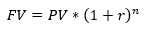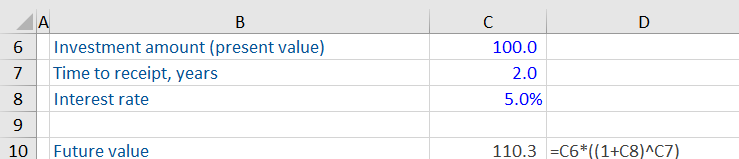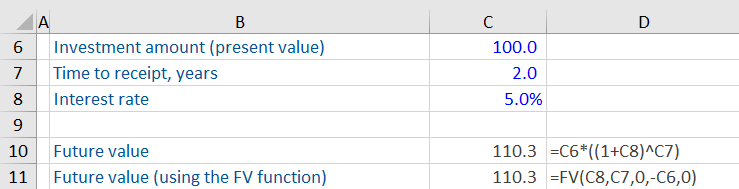## What is Future Value?

Future value (FV) is the value of an asset (e.g., a bond) at a future date based on a specified growth rate or rate of return. Future value helps investors estimate the value of an investment in the future. Future value is based on the concept of the time value of money (TVM). TVM has multiple applications in corporate finance, including stock and bond valuation, cost of capital, and capital budgeting.

## Key Learning Points

• Based on the concept of the time value of money, future value is the value of an asset or a stream of cash flows at a future date, based on a specified rate of return or growth rate
• Future value is calculated using the present value or current value of an investment, the rate of return expected by investors, and the number of periods needed for the investment
• Future value and present value have multiple applications in corporate finance, including in valuation, cost of capital, and capital budgeting
• Future value can be calculated using the future value formula or the FV function in Excel

## Time Value of Money

The time value of money says that future cash flows are worth less than immediate cash flows. We prefer immediate cash flows for many reasons, including:

• Cash now can be spent immediately rather than having to defer spending
• Cash now can be invested to give more cash in the future
• There is a risk of not receiving the future cash flow due to default

So, all else being equal, a cash flow now would be preferred over the same cash flow in the future. But being able to compare present and future cash flows of different amounts helps us determine in which situations we would be willing to wait for a future cash flow.

## Future Value Explained

If you deposit money with the bank, you would expect it to earn interest. And the value of your deposit would therefore be higher in one year’s time. We can calculate the future value using the formula below:• The present value (PV) represents today’s value
• r is the interest rate. Typically, the higher the risk, the higher the interest rate
• N represents the number of years the money will be kept in the account

## Example Calculation: Future Value

If you deposit \$100 at an interest rate of 5%, how much would you expect to have in two years?

### Using the Future Value Formula:

Based on the formula for calculating future value, we get the following:### Using the Future Value function in Excel:

We can also calculate the future value using the FV function in Excel:Where:

rate = Interest rate

nper = Number of years

pmt = Payments

pv = present value

type = Whether the payments are made at the beginning or the end of the period

The ‘pmt’ or payments function is useful when there are payments involved. For example, if an investment promises payments on a regular basis, such payments can increase the future value. In this case, there are no payments to investors as the interest is just accruing on the account, so we have kept ‘pmt’ as 0. ‘Type’ of 0 indicates that any cash flows happen at the end of each period.

Using the FV excel function, we get the same future value.